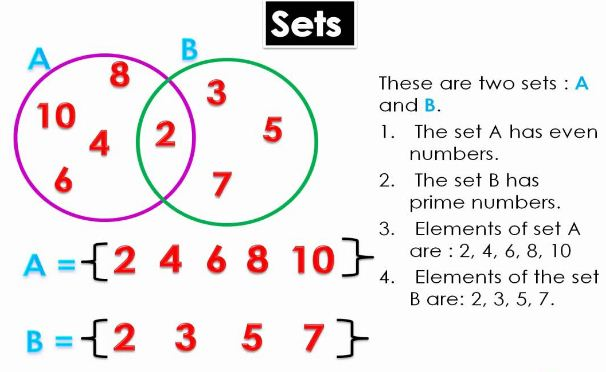## Introduction to Sets

A set is well defined class or collection of objects.A set is often described in the following two ways.

1. Roster method or Listing method: In this method a set is described by listing elements, separated by commas, within braces { }. The set of vowels of English alphabet may be described as {a, e, i, o, u}.
2. Set-builder method or Rule method: In this method, a set is described by a characterizing property P(x) of its elements x. In such a case the set is described by {x : P(x) holds} or {x | P(x) holds}, which is read as ‘the set of all x such that P(x) holds’. The symbol ‘|’ or ‘:’ is read as ‘such that’.
The set A = {0, 1, 4, 9, 16, ……} can be written as A = {x2 | x ∈ Z}.

### Symbols

 Symbol Meaning ⇒ Implies ∈ Belongs to A ⊂ B A is a subset of B ⇔ Implies and is implied by ∉ Does not belong to s.t.(: or |) Such that ∀ For every ∃ There exists iff If and only if & And a | b a is a divisor of b N Set of natural numbers I or Z Set of integers R Set of real numbers C Set of complex numbers Q Set of rational numbers

### Types of sets

1. Null set or Empty set: The set which contains no element at all is called the null set. This set is sometimes also called the ‘empty set’ or the ‘void set’. It is denoted by the symbol ϕ or { }.
2. Singleton set: A set consisting of a single element is called a singleton set. The set {5} is a singleton set.
3. Finite set: A set is called a finite set if it is either void set or its elements can be listed (counted, labelled) by natural number 1, 2, 3, … and the process of listing terminates at a certain natural number n (say).
Cardinal number of a finite set: The number n in the above definition is called the cardinal number or order of a finite set A and is denoted by n(A) or O(A).
4. Infinite set: A set whose elements cannot be listed by the natural numbers 1, 2, 3, …., n, for any natural number n is called an infinite set.
5. Equivalent set: Two finite sets A and B are equivalent if their cardinal numbers are same i.e. n(A) = n(B).
Example: A = {1, 3, 5, 7}; B = {10, 12, 14, 16} are equivalent sets, [∵ O(A) = O(B) = 4].
6. Equal set: Two sets A and B are said to be equal iff every element of A is an element of B and also every element of B is an element of A. Symbolically, A = B if x ∈ A ⇔ x ∈ B
Example: If A = {2, 3, 5, 6} and B = {6, 5, 3, 2}. Then A = B because each element of A is an element of B and vice-versa.
7. Universal set: A set that contains all sets in a given context is called the universal set.
It should be noted that universal set is not unique. It may differ in problem to problem.
8. Power set: If S is any set, then the family of all the subsets of S is called the power set of S.
The power set of S is denoted by P(S). Symbolically, P(S) = {T : T ⊆ S}. Obviously ϕ and S are both elements of P(S).
Example: Let S = {a, b, c}, then P(S) = {ϕ, {a}, {b}, {c}, {a, b}, {a, c}, {b, c}, {a, b, c}}.
Power set of a given set is always non-empty.

### Subsets

Subsets (Set inclusion): Let A and B be two sets. If every element of A is an element of B, then A is called a subset of B.
If A is subset of B, we write A ⊆ B, which is read as “A is a subset of B” or “A is contained in B”.
Thus, A ⊆ B ⇒ a ∈ A ⇒ a ∈ B.
Proper and improper subsets: If A is a subset of B and then A is a proper subset of B. We write this as ⊂.
The null set ϕ is subset of every set and every set is subset of itself, i.e. ϕ ⊂ A, A ⊆ A and for every set A. They are called improper subsets of A. Thus every non-empty set has two improper subsets. It should be noted that ϕ has only one subset ϕ which is improper.
All other subsets of A are called its proper subsets. Thus, if A ⊂ B, A ≠ B, A ≠ ϕ then A is said to be proper subset of B.
Example: Let A = {1,2}. Then A has ϕ; {1}, {2}, {1,2} as its subsets out of which ϕ and {1, 2} are improper and {1} and {2} are proper subsets.